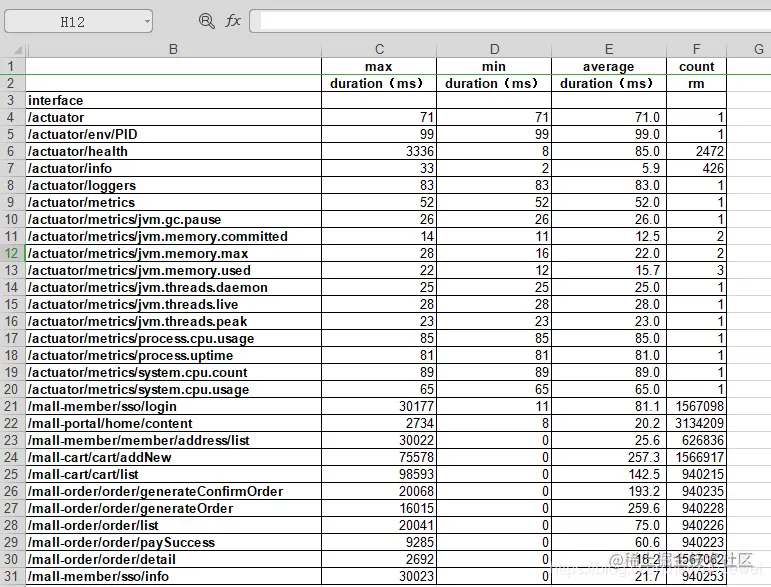# How to use Python to statistically analyze access logs?

2022-02-01 12:47:53 zuozewei

「 This is my participation 11 The fourth of the yuegengwen challenge 27 God , Check out the activity details ：2021 One last more challenge

## One 、 Preface

Business modeling in performance scenarios is a very important part of performance testing . And in our real project , There are too many cases where business models are different from online business models . There may be a variety of reasons , These reasons greatly reduce the value of performance testing .

In today's article , What I want to write is the simplest logic . That's from gateway based access Log statistical analysis is transformed into a general business model in a specific scenario . Please refer to 《 Performance test practice 30 speak 》 Medium 【14 Performance test scenarios ： How to understand the business model ？】

## Two 、 Front work

First, we take the gateway of the day from the peak day access journal , Here are some examples 1400+ Ten thousand records

``````[[email protected] ~]# wc -l access.log
14106419 access.log
Copy code ``````

As for gateway access How to configure logs , See the previous article SpringCloud Two or three things of log in pressure test

What we got access The content of the log is generally as follows ：

``````10.100.79.126 - - [23/Feb/2021:13:52:14 +0800] "POST /mall-order/order/generateOrder HTTP/1.1" 500 133 8201 52 ms
Copy code ``````

The corresponding fields are as follows ：

``````address, user, zonedDateTime, method, uri, protocol, status, contentLength, port, duration.
Copy code ``````

that , Here comes our need , How to analyze access journal , Get the maximum processing time of each interface gateway 、 minimum value 、 Average and visits .

Here I extend the statistical analysis to get the processing time of each interface gateway , Facilitate the performance evaluation of our interface .

## 3、 ... and 、 To write Python Scripts complete data analysis

We know that in data analysis 、 In the field of machine learning, it is generally recommended to use Python, Because this is Python What you are good at . And in the Python In data analysis ,Pandas The frequency of using is very high , If our daily data processing work is not very complicated , You usually use a few words Pandas The code can regulate the data . So here we just need to put it in the log duration Fields are stored in pandas The basic data structure of DataFrame in , And then by grouping 、 Data statistics function can be realized .

The whole project includes 4 Parts of ：

• The first part is data loading , First we pass open File read data loaded into memory . Note that when the log file is large, do not use readlines()、readline(), Will read all logs to memory , Cause memory to be full . So use it here `for line in fo` Iterative approach , Basically no memory ;
• The second step is data preprocessing . Read log file , have access to `pd.read_table(log_file, sep=’ ‘, iterator=True)`, But here we set up sep Cannot match segmentation properly , So first use split Division , And then deposit pandas;
• The third step is data analysis ,Pandas Provides IO The tool can read large files in blocks , Use different block sizes to read and then call `pandas.concat` Connect `DataFrame`, And then use Pandas Common statistical function analysis ;
• The last step is data loading , Save the statistical analysis results to Excel In file .

``````#pip3 install  Package name  -i  The source of url  Temporary source change
# Tsinghua University source ：https://pypi.tuna.tsinghua.edu.cn/simple/

#  Powerful data structure library , For data analysis , Time series and statistics, etc
pip3 install pandas -i https://pypi.tuna.tsinghua.edu.cn/simple/

#  Handle  URL  My bag
pip3 install urllib -i https://pypi.tuna.tsinghua.edu.cn/simple/

#  Install build execl Related modules of the form
pip3 install xlwt -i https://pypi.tuna.tsinghua.edu.cn/simple/
Copy code ``````

The specific code is as follows ：

``````# Count the processing time of each interface
import sys
import os
import pandas as pd
from urllib.parse import urlparse
import re

'''  Global parameter  '''
mulu=os.path.dirname(__file__)
# Log file storage path
logdir="D:\log"
# Store the log related fields required for statistics
logfile_format=os.path.join(mulu,"access.log")

for eachfile in os.listdir(logdir):
logfile=os.path.join(logdir,eachfile)
with open(logfile, 'r') as fo:
for line in fo:
spline=line.split()
# Filter the exception part of the field
if spline=="-":
pass
elif spline=="GET":
pass
elif spline[-1]=="-":
pass
else:
# It can be interpreted as url Address
parsed = urlparse(spline)
# print('path :', parsed.path)
# Exclude numerical parameters
interface = ''.join([i for i in parsed.path if not i.isdigit()])
# print(interface)
# Rewrite file
with open(logfile_format, 'a') as fw:
fw.write(interface)
fw.write('\t')
fw.write(spline[-2])
fw.write('\n')
print ("output panda")

'''  Data analysis  '''
# Read the statistical field into dataframe in
loop=True
chunksize=10000000
chunks=[]
while loop:
try:
chunks.append(chunk)
except StopIteration:
loop=False
print ("Iteration is stopped.")

df=pd.concat(chunks)
#df=df.set_index("interface")
#df=df.drop(["GET","-"])

df_groupd=df.groupby('interface')
df_groupd_max=df_groupd.max()
df_groupd_min= df_groupd.min()
df_groupd_mean= df_groupd.mean()
df_groupd_size= df_groupd.size()

df_ana=pd.concat([df_groupd_max,df_groupd_min,df_groupd_mean,df_groupd_size],axis=1,keys=["max","min","average","count"])
print ("output excel")
df_ana.to_excel("result.xls")
Copy code ``````

Running results ：In this way, we can easily get the business volume statistics and interface processing time on peak days .

## Four 、 Summary

Through today's example, we should be able to see the adoption of Python For performance engineers, it reduces the technical threshold of data analysis . I believe in today's DT Time , Any position needs to use the thinking and ability of data analysis .

In this paper, the source code ：

Reference material ：